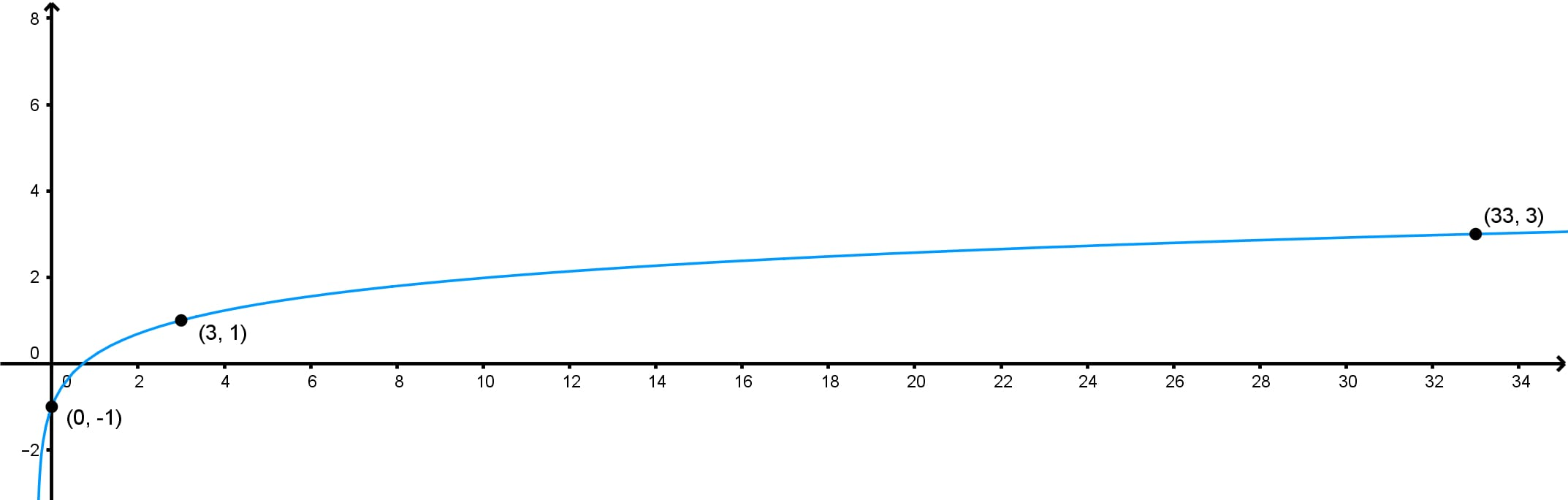# Finding a logarithmic function given its graph#### Everything You Need in One Place

Homework problems? Exam preparation? Trying to grasp a concept or just brushing up the basics? Our extensive help & practice library have got you covered.#### Learn and Practice With Ease

Our proven video lessons ease you through problems quickly, and you get tonnes of friendly practice on questions that trip students up on tests and finals.#### Instant and Unlimited Help

Our personalized learning platform enables you to instantly find the exact walkthrough to your specific type of question. Activate unlimited help now!##### Intros
###### Lessons
1. Introduction to finding logarithmic function given its graph

i. What is a logarithmic function?

ii. How can we determine the equation of a logarithmic graph?

##### Examples
###### Lessons
1. Determining the Equation of an Exponential Function Given Its Graph

Determine an exponential function in the form $y = \log_{b} x$ with the given graph.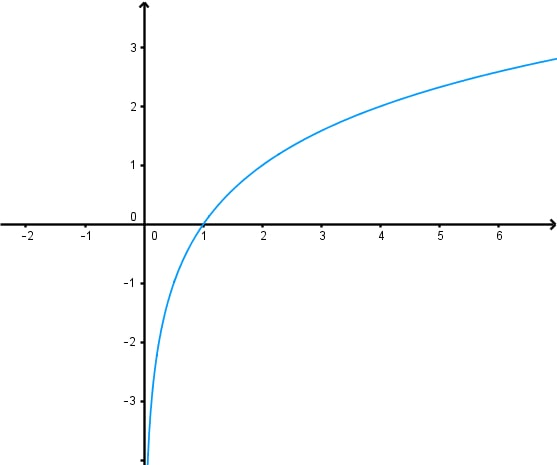1. Determining the Equation of a Transformed Logarithmic function given its Graph

Determine a logarithmic function in the form $y = \log_{2} (x + b) + c$ for each of the given graphs.

1.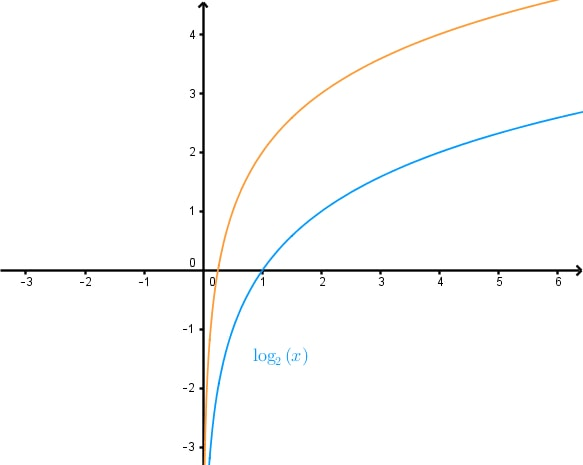2.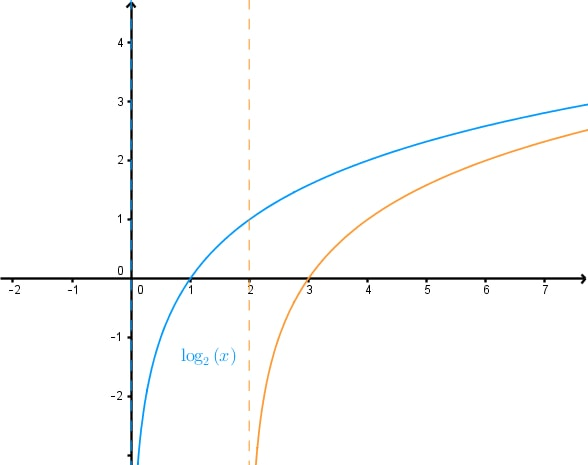2. Determining the Equation of a Transformed Logarithmic function given its Graph - Continued

Determine a logarithmic function in the form $y = A \log_{2} (Bx)$ for each of the given graphs.

1.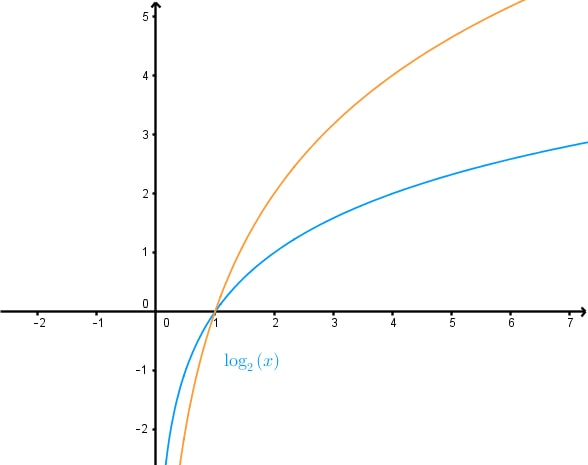2.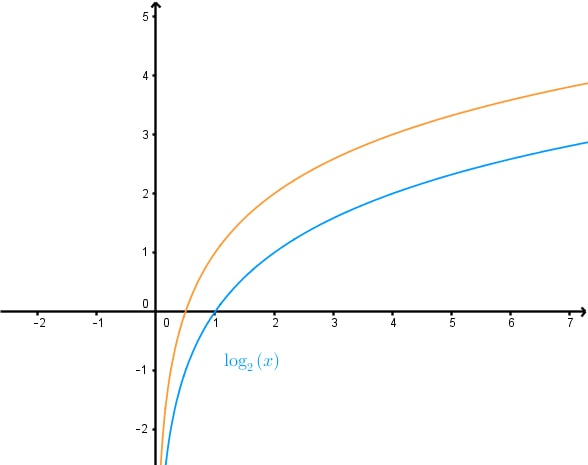3.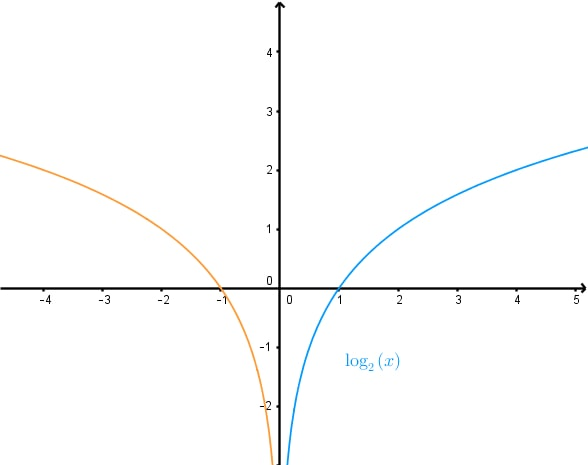4.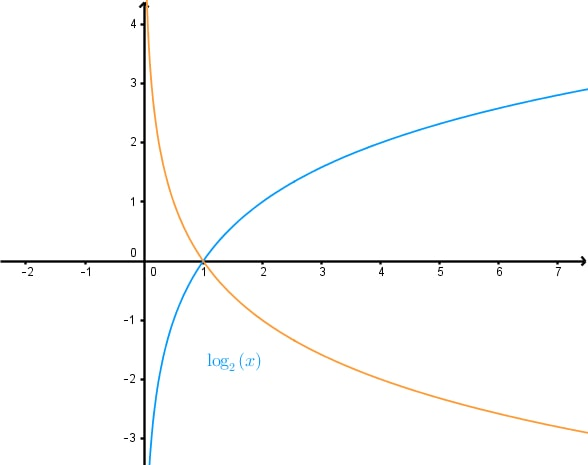3. Determining the Equation of a Logarithmic Graph With Multiple Transformations

Determine a logarithmic function in the form $y = A \log (Bx+1)+C$ for each of the given graphs.